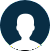#### You have questions? We got answers!Calculus Intergrals Help?

Science & Mathematics by Anonymous 2018-09-20 22:19:14

Social Science

Calculus Intergrals Help?

No response

1) What is an integrals that will find the volume of the solid that is formed when the region bounded by the graphs of y = e^2x, x = 1, and y = 1 is revolved around the line y = -1. 2) Find the average value of f(x) equals 1/x over the interval [1, 3]. 3) Determine if the Mean Value Theorem for Integrals...

ReCapcha
Not a bot